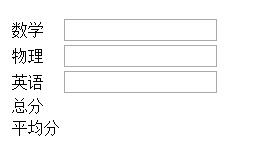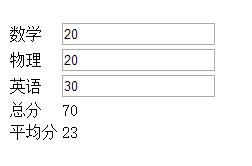# Vue.js的简单自动求和计算实例_冘醉_前端开发者

<script src=”https://cdn.bootcss.com/vue/2.5.16/vue.js”></script>

```<div id="vuetest">
<table>
<tr>
<td>数学</td>
<td><input type="text" v-model.number="math"></td>
</tr>
<tr>
<td>物理</td>
<td><input type="text" v-model.number="physics"></td>
</tr>
<tr>
<td>英语</td>
<td><input type="text" v-model.number="english"></td>
<tr>
<td>总分</td>
<td>{{sum}}</td>
</tr>
<tr>
<td>平均分</td>
<td>{{average}}</td>
</tr>
</tr>
</table>
</div>```1.创建一个 vue容器 在el中写你的容器id。

2.在data中写你的数据值，注意与html页面中的v-model.number=中写的相对应。

（v-model是负责监听用户的输入事件以更新数据，并对一些极端场景进行一些特殊处理）

math：后面也可以写固定的值像math:“90”,以此类推

3.绑定你的表达式，用computed监控前面定义的变量并写一个方法。

4.在文本框里输入数字就可以自动进行动态的求和，平均值计算了，是不是很方便。

js代码如下

```var vuetest = new Vue({
el:'#vuetest',
data:{
math:'',
physics:'',
english:'',
},
computed :{
sum:function () {
return parseFloat(this.math)+this.physics+this.english;
},
average :function(){
return Math.round(this.sum/3);
}
}
});```» 本文链接地址：https://www.rokub.com/71272.html
» 您也可以订阅本站：https://www.rokub.com
64K

• 回顶